Ex 6.3

Chapter 6 Class 12 Application of Derivatives
Serial order wise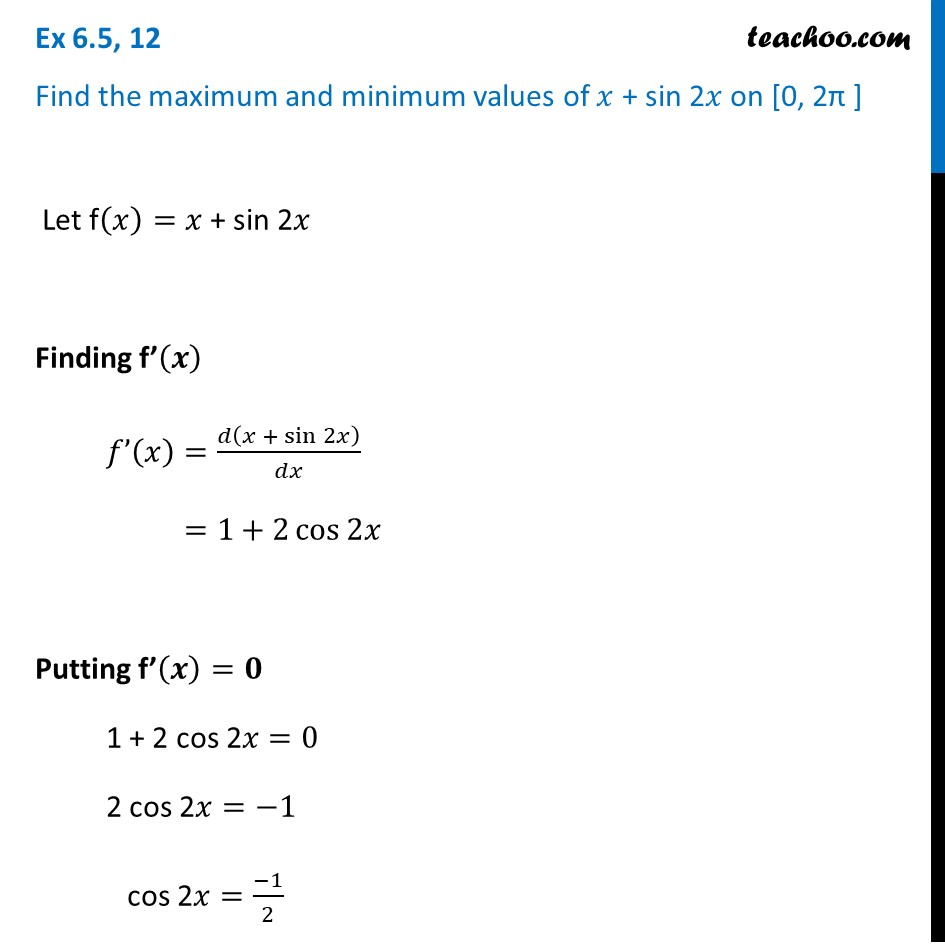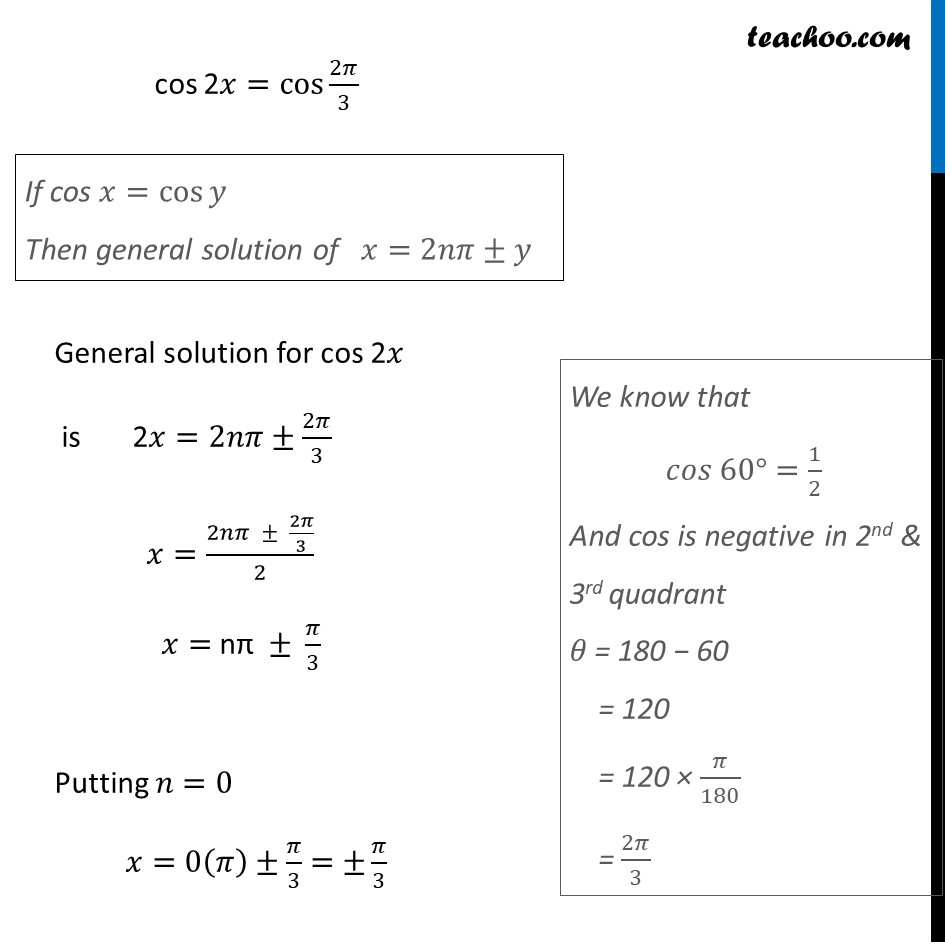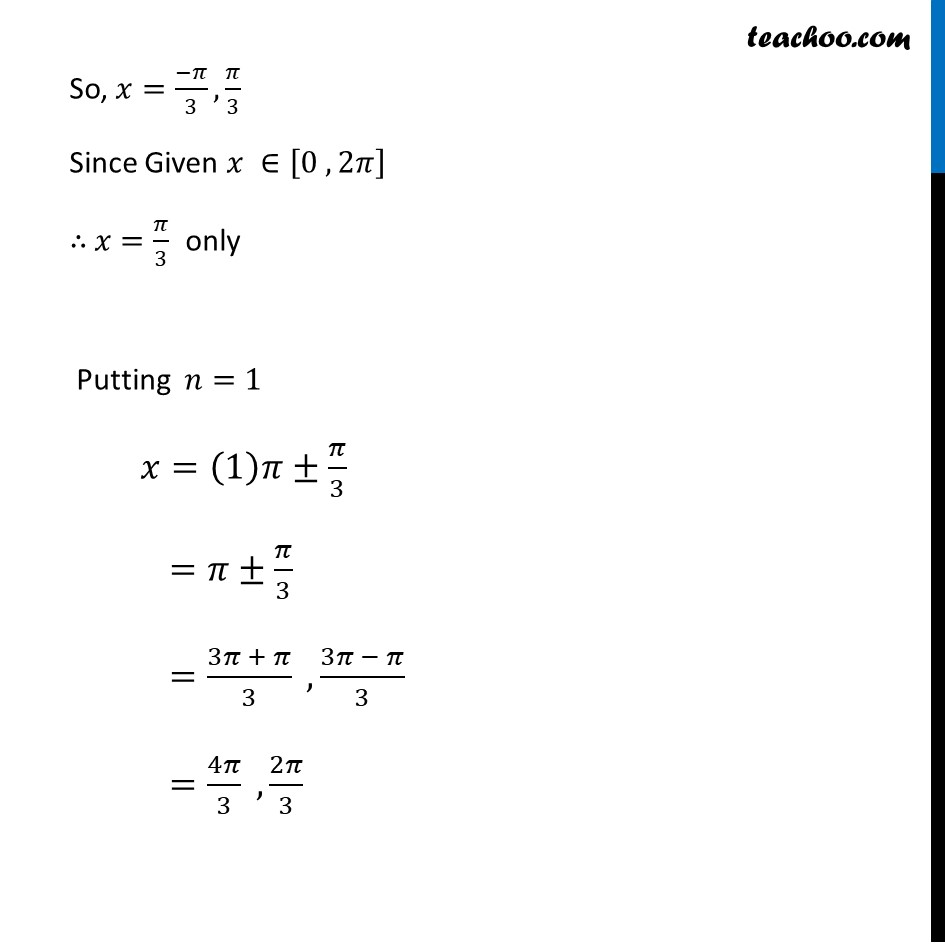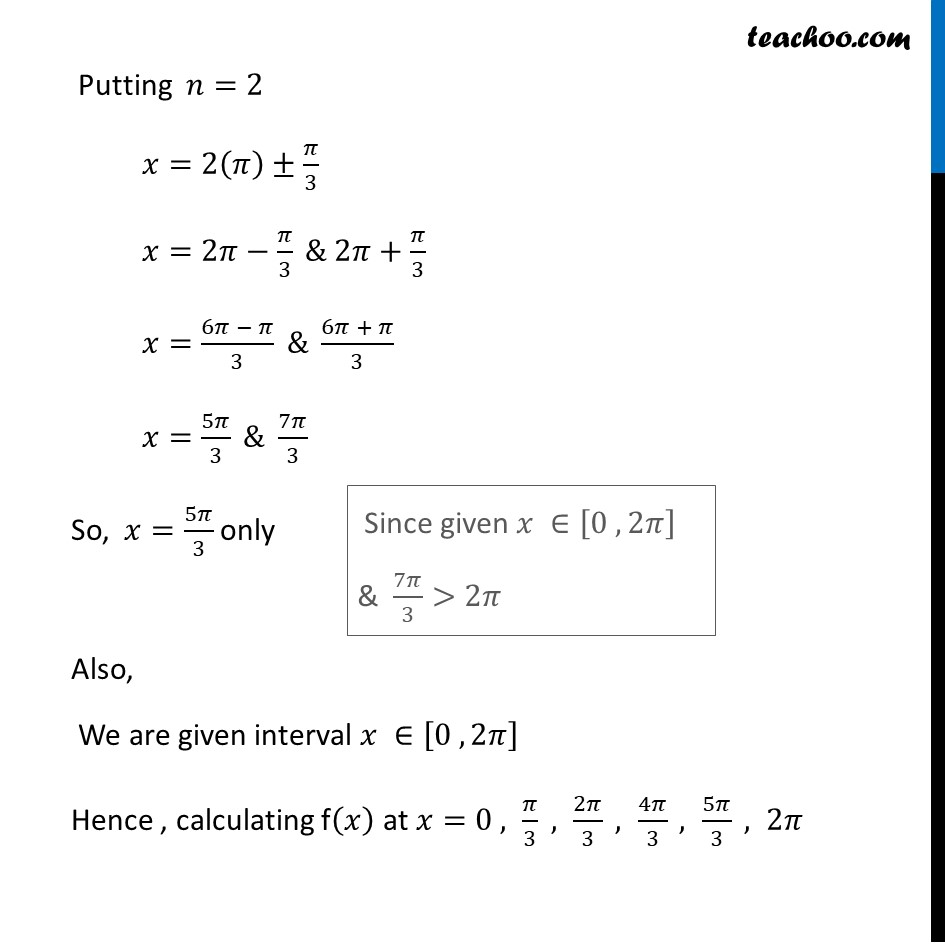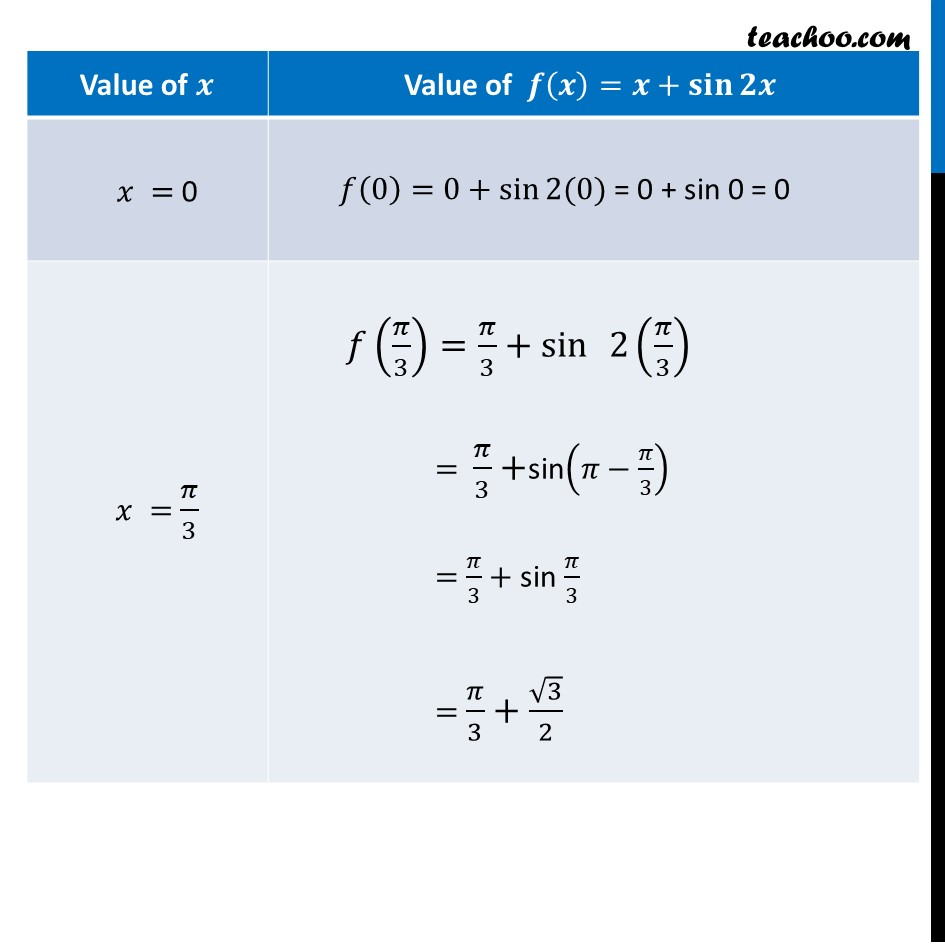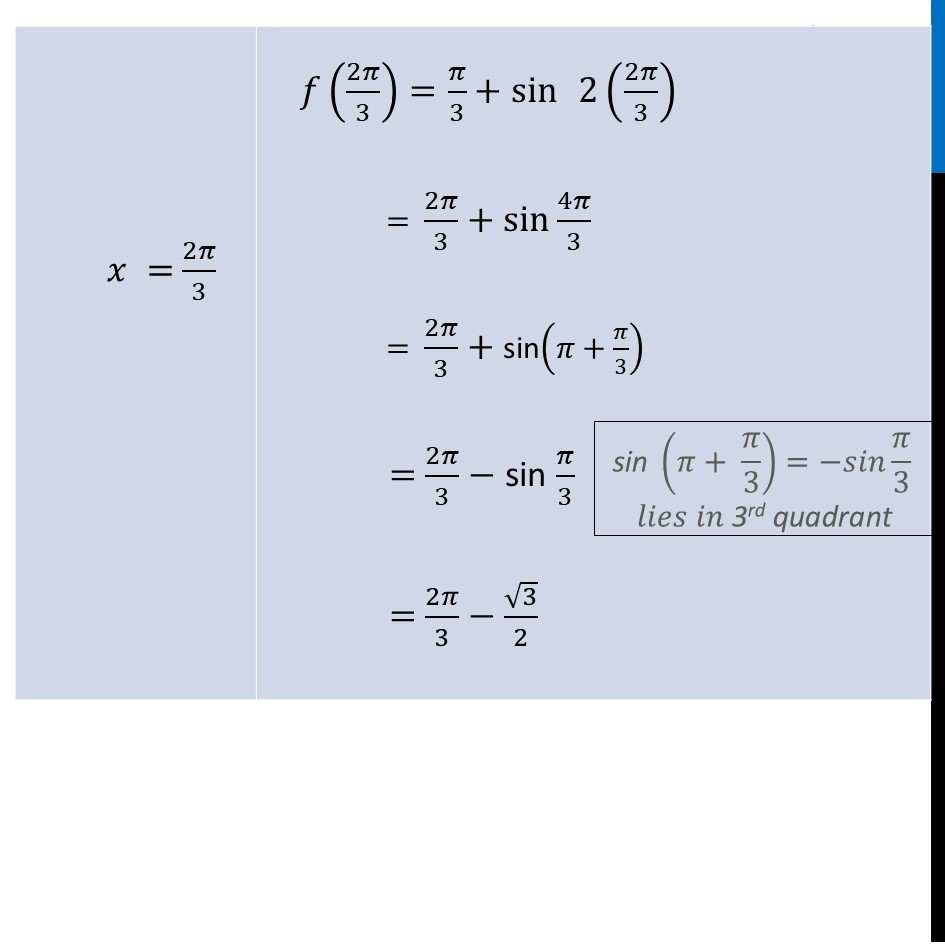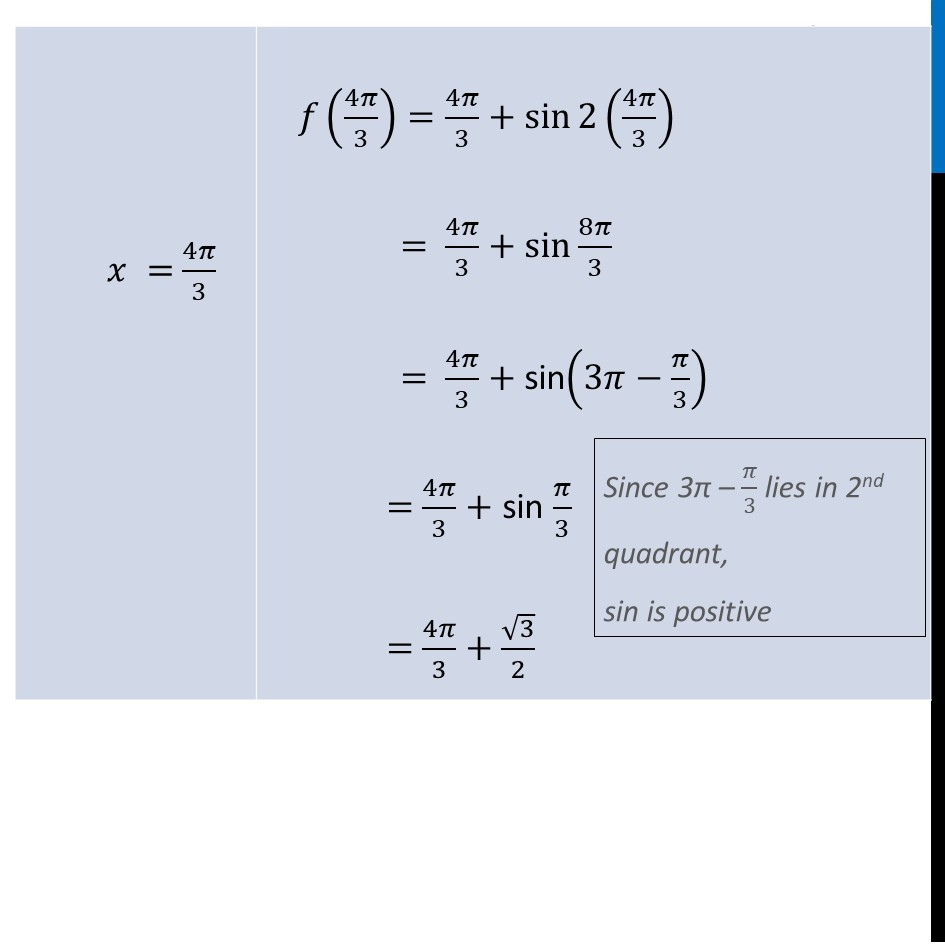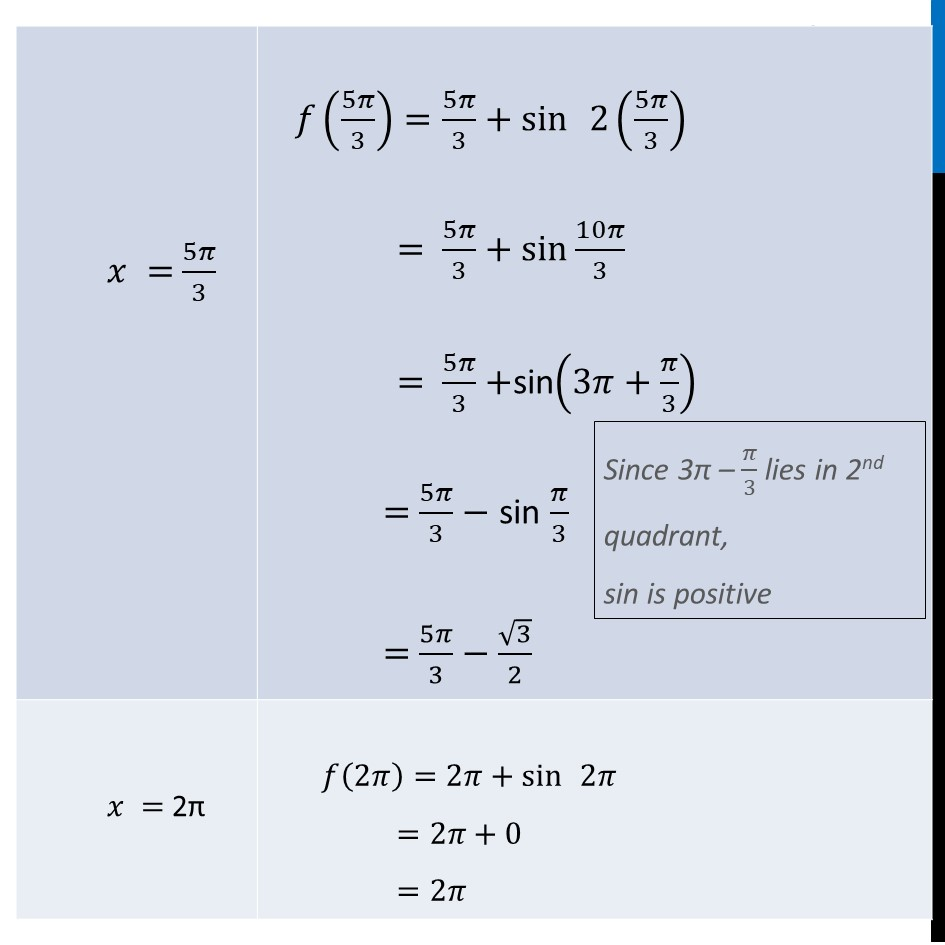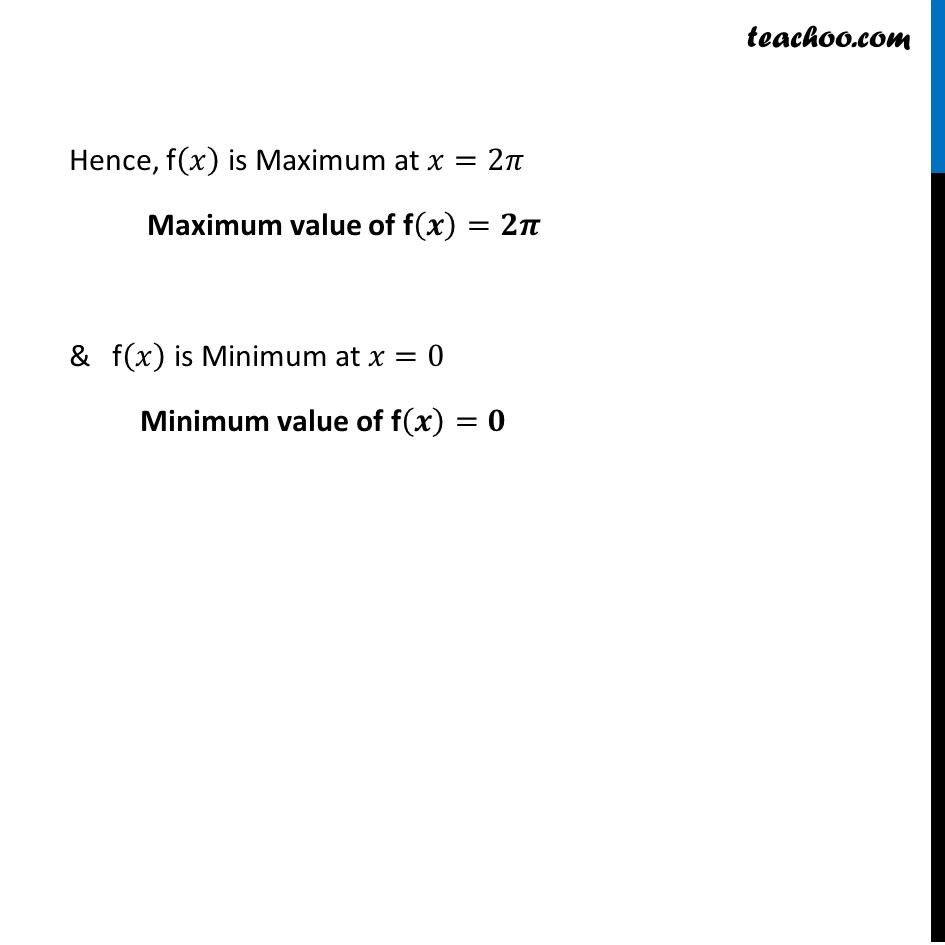Learn in your speed, with individual attention - Teachoo Maths 1-on-1 Class

### Transcript

Ex 6.3, 12 Find the maximum and minimum values of 𝑥 + sin 2𝑥 on [0, 2π ] Let f(𝑥)=𝑥 + sin 2𝑥 Finding f’(𝒙) 𝑓’(𝑥)=𝑑(𝑥 + sin 2𝑥)/𝑑𝑥 =1+2 cos⁡2𝑥 Putting f’(𝒙)=𝟎 1 + 2 cos 2𝑥=0 2 cos 2𝑥=−1 cos 2𝑥=(−1)/2 cos 2𝑥=cos⁡〖2𝜋/3〗 General solution for cos 2𝑥 is 2𝑥=2𝑛𝜋±2𝜋/3 𝑥=(2𝑛𝜋 ± 2𝜋/3)/2 𝑥= nπ ± 𝜋/3 Putting 𝑛=0 𝑥=0(𝜋)±𝜋/3 =±𝜋/3 " " cos 2𝑥=cos⁡〖2𝜋/3〗 General solution for cos 2𝑥 is 2𝑥=2𝑛𝜋±2𝜋/3 𝑥=(2𝑛𝜋 ± 2𝜋/3)/2 𝑥= nπ ± 𝜋/3 Putting 𝑛=0 𝑥=0(𝜋)±𝜋/3 =±𝜋/3 " " We know that 𝑐𝑜𝑠 60°=1/2 And cos is negative in 2nd & 3rd quadrant 𝜃 = 180 − 60 = 120 = 120 × 𝜋/180 = 2𝜋/3 So, 𝑥=(−𝜋)/3,𝜋/3 Since Given 𝑥 ∈[0 , 2𝜋] ∴ 𝑥=𝜋/3 only Putting 𝑛=1 𝑥=(1)𝜋±𝜋/3 =𝜋±𝜋/3 =(3𝜋 + 𝜋)/3 , (3𝜋 − 𝜋)/3 =4𝜋/3 , 2𝜋/3 Putting 𝑛=2 𝑥=2(𝜋)±𝜋/3 𝑥=2𝜋−𝜋/3 & 2𝜋+𝜋/3 𝑥=(6𝜋 − 𝜋)/3 & (6𝜋 + 𝜋)/3 𝑥=5𝜋/3 & 7𝜋/3 So, 𝑥=5𝜋/3 only Also, We are given interval 𝑥 ∈[0 , 2𝜋] Hence , calculating f(𝑥) at 𝑥=0 , 𝜋/3 , 2𝜋/3 , 4𝜋/3 , 5𝜋/3 , 2𝜋 Since given 𝑥 ∈[0 , 2𝜋] & 7𝜋/3>2𝜋 𝑓(2𝜋/3)=𝜋/3+sin⁡ 2(2𝜋/3) = 2𝜋/3+sin⁡〖4𝜋/3 〗 = 2𝜋/3+ sin(𝜋+𝜋/3) = 2𝜋/3− sin 𝜋/3 = 2𝜋/3−√3/2 𝑓(4𝜋/3)=4𝜋/3+sin⁡2 (4𝜋/3) = 4𝜋/3+sin⁡〖8𝜋/3 〗 = 4𝜋/3+ sin(3𝜋−𝜋/3) = 4𝜋/3+ sin 𝜋/3 = 4𝜋/3+√3/2 𝑓(4𝜋/3)=4𝜋/3+sin⁡2 (4𝜋/3) = 4𝜋/3+sin⁡〖8𝜋/3 〗 = 4𝜋/3+ sin(3𝜋−𝜋/3) = 4𝜋/3+ sin 𝜋/3 = 4𝜋/3+√3/2 Since 3π – 𝜋/3 lies in 2nd quadrant, sin is positive 𝑓(5𝜋/3)=5𝜋/3+sin⁡ 2(5𝜋/3) = 5𝜋/3+sin⁡〖10𝜋/3 〗 = 5𝜋/3+sin(3𝜋+𝜋/3) = 5𝜋/3− sin 𝜋/3 = 5𝜋/3−√3/2 Since 3π – 𝜋/3 lies in 2nd quadrant, sin is positive 𝑓(2𝜋)=2𝜋+sin⁡ 2𝜋 =2𝜋+0 =2𝜋 Hence, f(𝑥) is Maximum at 𝑥=2𝜋 Maximum value of f(𝒙)=𝟐𝝅 & f(𝑥) is Minimum at 𝑥=0 Minimum value of f(𝒙)=𝟎